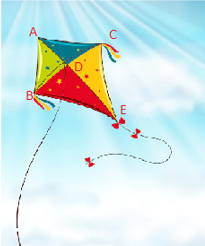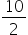Mathematics
Easy

Question

#ABCED is a kite, AB = 5x – 12, AC = 3x – 2 , BE = 4x , CE = 7x -15 , DC = 10 units, AB, AC, BE, CE, DC are line segmentFind AB.

## 10111213Hint:

## The correct answer is: 13

### AB = 5x  - 12 AC = 3x - 2 By perpendicular bisector theorem.AB = AC5x – 12 = 3x -22x = 12 – 2 x =x = 5AB = 5*5 – 12 = 25 – 12 = 13

Pythagoras theorem states that “In a right-angled triangle, the square of the hypotenuse side is equal to the sum of squares of the other two sides“. T

### Related Questions to study#### With Turito Foundation.#### Get an Expert Advice From Turito.